Learning Library

Fun Math Facts

How do you make learning addition and subtraction facts fun? Try your hand at "popping" colorful balloons with equations that equal one!

Click on a worksheet in the set below to see more info or download the PDF.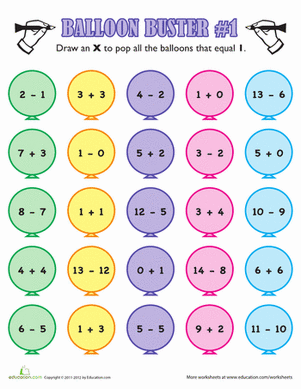Fun Math Facts

How do you make learning addition and subtraction facts fun? Try your hand at "popping" colorful balloons with equations that equal one!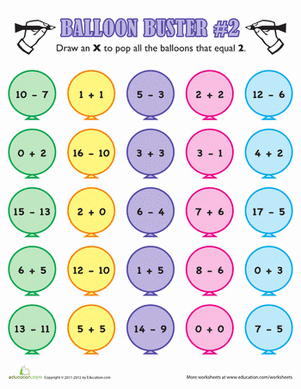Balloon Buster #2

Little math learners, see how many math fact balloons you can pop! This is a great review of simple addition and subtraction equations.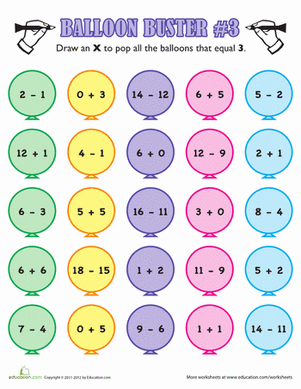Balloon Buster #3

Get a fun review of math facts, and try your hand at "popping" colorful balloons that have an addition or subtraction equation that equals the number three.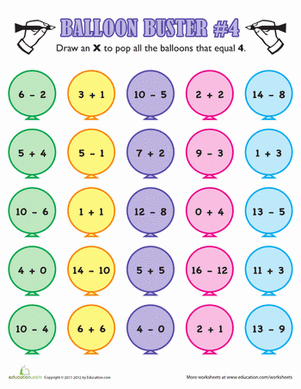Balloon Buster #4

Get your little one excited about math practice with a fun game of balloon buster! She'll get a great review of addition and subtraction facts.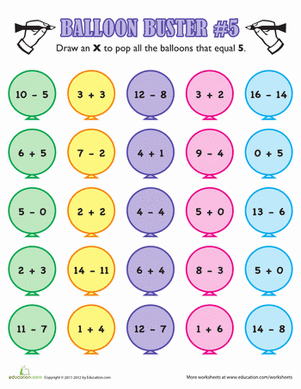Balloon Buster #5

Need help with simple addition and subtraction? Check out balloon busters, a fun game where your little one can "pop" balloons with the right equation!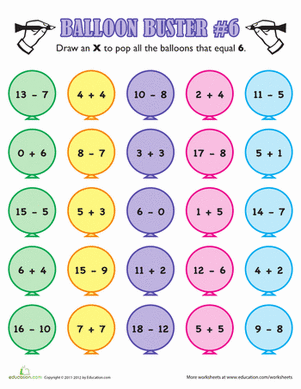Balloon Buster #6

How do you make learning addition and subtraction facts fun? Try your hand at "popping" colorful balloons with equations that equal six!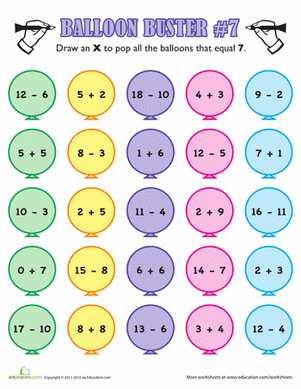Balloon Buster #7

Little math learners, see how many math fact balloons you can pop! With this game, your child will review simple addition and subtraction equations.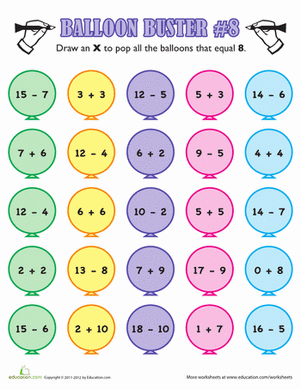Balloon Buster #8

Does your child have her math facts memorized? Help her review simple addition and subtraction the fun way, with a game of balloon buster!Balloon Buster #9

Help your little one get excited about math practice with a fun game of balloon buster! She'll get a great review of addition and subtraction facts.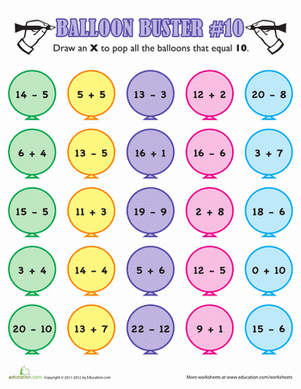Balloon Buster #10

This balloon busters game gets kids to do mental math in search of the number 10. Kids will 'pop' each balloon that equals 10 in this fun math worksheet.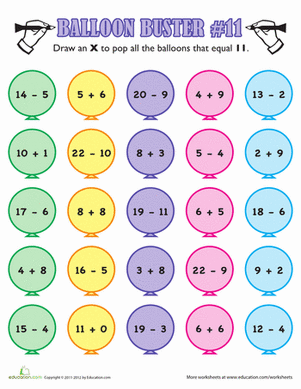Balloon Buster #11

How do you make learning addition and subtraction facts fun? Try your hand at "popping" colorful balloons showing a sum or difference of eleven!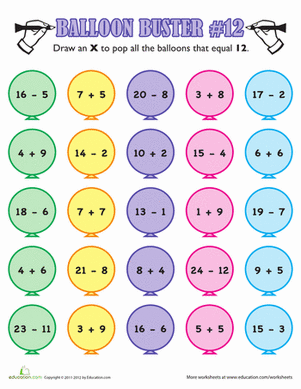Balloon Buster #12

Little math learners, see how many math fact balloons you can pop! Your child will get a great review of simple addition and subtraction equations.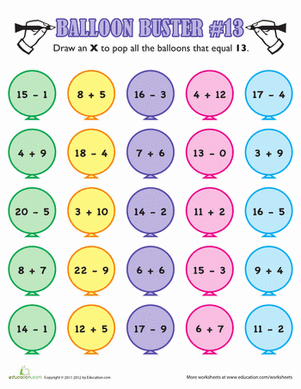Balloon Buster #13

Get a fun review of math facts, and try your hand at "popping" colorful balloons with equations that equal the number thirteen.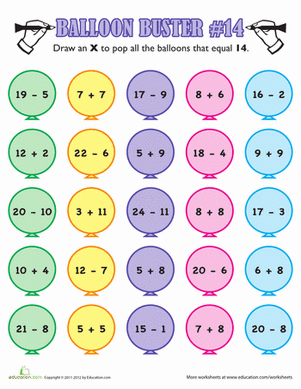Balloon Buster #14

Put some 'pop' in your math review with this balloon buster worksheet! Kids will use their mental math skills to find equations that equal 14.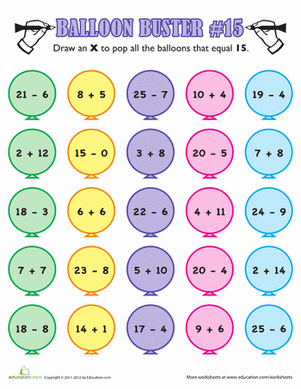Balloon Buster #15

Get a satisfying 'pop' out of math with this balloon-themed worksheet. Kids will draw an 'X' to 'pop' any balloons that equal 15.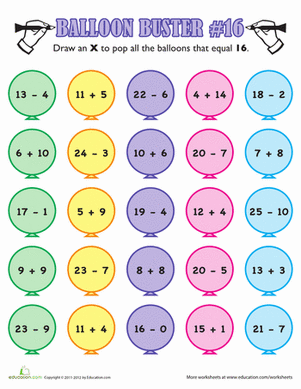Balloon Buster #16

How do you make learning addition and subtraction facts fun? Try your hand at "popping" colorful balloons with a sum or difference of sixteen.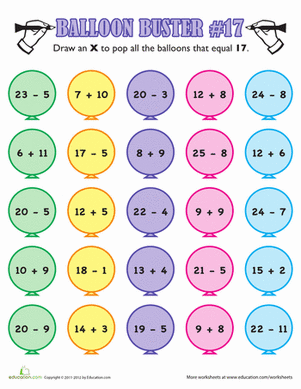Balloon Buster #17

Little math learners, see how many math fact balloons you can pop! Your child will review one and two-digit addition and subtraction equations.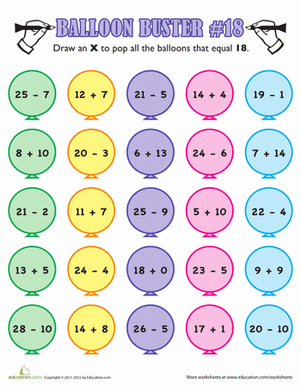Balloon Buster #18

Get a fun review of math facts, and try your hand at "popping" colorful balloons with equations that equal the number eighteen.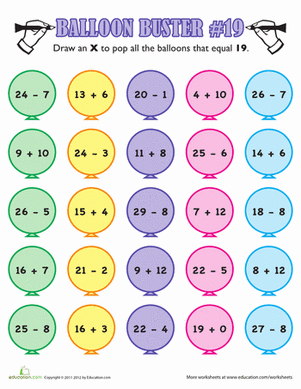Balloon Buster #19

'Pop' the balloons with equations that equal 19 in this fun mental math worksheet. Have fun and learn math with this balloon buster game.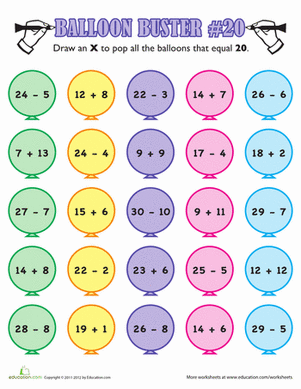Balloon Buster #20

Does your little mathematician enjoy the pop of a good balloon? In this sheet, he'll 'pop' balloons that contain addition or subtraction problems that equal 20.

Want to download this whole set as a single PDF?

Create new collection

0

New Collection>

0 items

What could we do to improve Education.com?

Please note: Use the Contact Us link at the bottom of our website for account-specific questions or issues.

What would make you love Education.com?